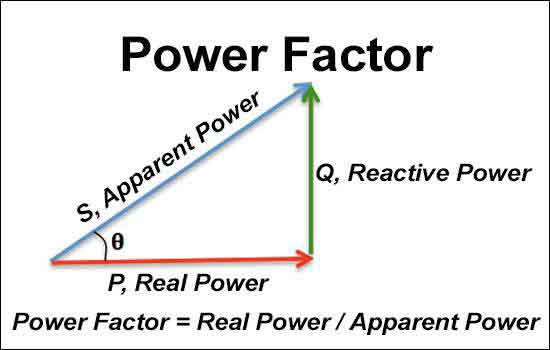# How to Calculate Power Factor

#### By R.W. Hurst, EditorHow to Calculate Power Factor

Calculating power factor (PF) is a straightforward process that requires measuring real power (RP) (true power) and apparent power (AP) and then using the PF formula. PF correction can be used to improve the PFof an electrical circuit, and the PF triangle can be used to visualize the relationship between RP, reactive power, and AP.

In an electrical circuit, power is a vital factor to consider. Power is the rate at which energy is transferred or converted and can be measured in watts. In
ac circuits the PF can be further categorized into types such as RP, reactive power, and AP. However, one important aspect of power often overlooked is the PF. PF is the ratio of RP to AP, measured in a range of values from 0 to 1. A poor PF can lead to energy waste, while a good PF can reduce energy consumption and improve efficiency.

PF Formula

To calculate PF, we must first understand the concepts of real, reactive, and AP. Real power (P) is the power consumed by the circuit and measured in watts. Reactive power (Q) is the power that oscillates back and forth between the source and resistive load without being consumed, and it is measured in volt-amperes reactive (VAR). Apparent power (S) is the total power supplied to the circuit, measured in volt-amperes (VA). The PF is the ratio of RP to AP, represented by the symbol "PF."

The PF formula is:

PF = P/S

Alternatively, we can also use the formula:

PF = cos(θ)

Where θ is the phase angle between the voltage and current.

The PF ranges from 0 to 1, with 1 being the ideal value. A PF of 1 indicates that all the power supplied to the circuit is being used, while a PF of 0 indicates that none of the power is being used.

Power Factor Calculation

PF can be calculated using the following formula:

PF = RP / AP

This formula can be rearranged to calculate any of the three components of the power triangle. For example, to calculate RP, the formula becomes:

Real power = Apparent power x Power factor

To calculate reactive power, the formula becomes:

Reactive power = Apparent power x sin (phase angle)

where the phase angle is the angle between the voltage and current waveforms.

The easiest way to calculate PF is by using a power meter directly measuring the real and AP. PF can be read off the meter display or calculated manually using the above formula.

The Easiest Way to Calculate Power Factor

To calculate PF, we need to measure the circuit's RP and AP. Measuring RP is straightforward, as it can be done using a wattmeter. However, measuring AP requires a bit more effort, as it involves measuring the voltage and current of the circuit and then multiplying them together. The easiest way to measure AP is by using a power meter or power analyzer, which can simultaneously measure both real and AP.

Once we have measured both real and apparent power, we can plug them into the PF formula and calculate the PF. For example, if a circuit has a RP of 500 watts and an apparent power of 600 VA, the PF can be calculated as follows:

PF = P/S = 500/600 = 0.83

Therefore, the PF of the circuit is 0.83.

Calculating 3-Phase Power Factor

In a 3-phase power system, PF be calculated similarly to a single-phase system. However, there are some additional considerations to take into account. In a 3-phase system, there are three voltage and current phases, each with its own PF. The overall PF of the system is the average of the three individual power factors.

To calculate the PF of a 3-phase system, we need to measure the total RP and the total AP of the system. We can do this by measuring the voltage and current of each phase and then using the following formulas:

Total real power (P) = √3 x voltage x current x power factor

Total apparent power (S) = √3 x voltage x current

Once we have measured the total RP and total AP, we can calculate the PF using the formula:

PF = P/S

For example, if a 3-phase system has a total RP of 1500 watts and a total apparent power of 1800 VA, the PF can be calculated as follows:

PF = P/S = 1500/1800 = 0.83

Therefore, the PF of the 3-phase system is 0.83.

Poor Power Factor

A poor PF can lead to energy waste, increased costs, and decreased efficiency. PF correction is the process of improving the PF of an electrical circuit by adding capacitors or other devices. Capacitors can provide reactive power that cancels out the reactive power of the load, leading to a higher PF. PF correction can be instrumental in industrial and commercial applications where large amounts of power are consumed.

Power Factor Triangle

The relationship between real, reactive, and AP can be visualized using the PF triangle. The PF triangle is a diagram that shows the relationship between the three types of power and the PF. In the PF triangle, RP is represented by the horizontal leg, the vertical leg represents reactive power, and the hypotenuse represents AP. The angle between the horizontal leg and the hypotenuse represents the phase angle and is equal to the arccosine of the PF.

• 1
• 2
• 3
• 4
• 5
• 6
• 7
• 8
• 9
• 10
• 11
• 12
• 13
• 14
• 15
• 16
• 17
• 18
• 19
• 20
• 21
• 22
• 23
• 24
• 25
• 26
• 27
• 28
• 29
• 30
• 31

Top